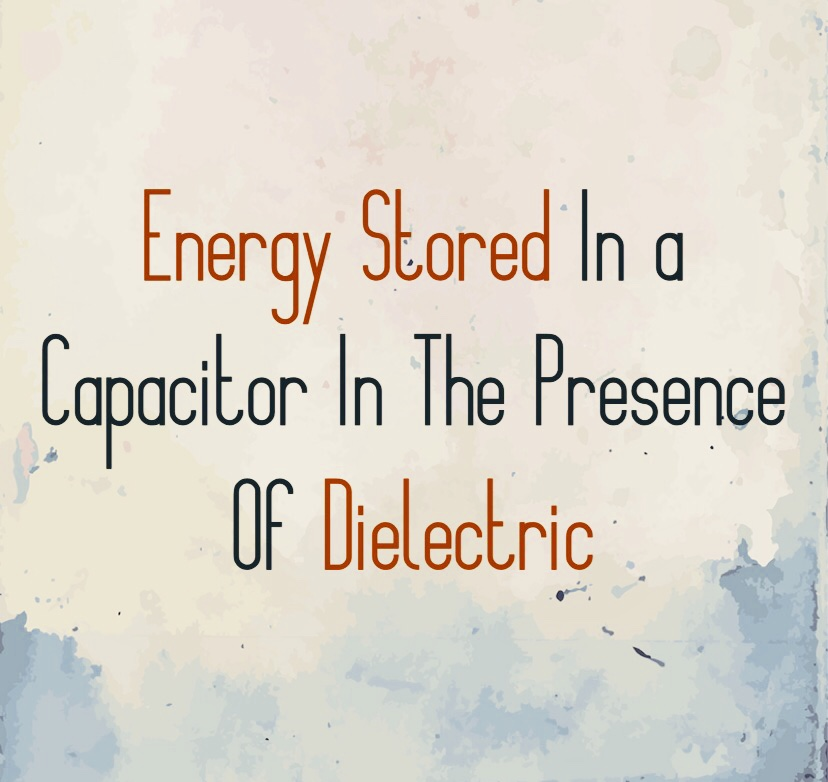## Energy Stored In a Capacitor In The Presence Of DielectricEnergy Stored In a Capacitor In The Presence Of Dielectric

The energy stored in a capacitor with a dielectric is given as

U=1/2 (CV)²  → 1

Here C = Capacitance = ∈ A/d

And V = E.d

Putting the above values in Equation.1

U = 1/2 (∈A/d ) (E.d)²

U = 1/2 ∈ A/d E². d²

U = 1/2 ∈ A/d E² (A. d) ⟶ 2

In the above equation, A.d is the volume between the plates of the capacitor containing the electric field and ∈E²  = k∈∘E²

= k∈∘

So,
U/A.d = 1/2× k×∈∘×E²

In the above equation, U/A.d is the energy per unit volume.

U/A.d = Uₑ

So,
Uₑ = 1/2×∈∘×E²

The above equation gives the energy stored per unit volume in a capacitor with a dielectric. It clearly shows that energy stored per unit volume in a capacitor with a dielectric is proportional to the square of the electric field.

## Numerical:

Two parallel plate capacitors having capacitances C₁ = C₂ = 2μF are connected in a parallel across a 12 volts voltage source (battery).

To Find
(a). The charge on each capacitor
(b). The total energy stored in the capacitor.

(a).

As
Q = CV

Q = (2μF)(12 volts)

Q = 24μC

Since capacitors are connected in parallel to the charge on each capacitor will be the same.

(b).

Energy stored in each of the capacitors can be calculated using the relation
U=1/2×C×V²

U=1/2×(2μF)×(12V)²

U=144μJ

Since there are two capacitors so the total energy on the combination can be written as

Uₜₒₜₐₗ = 2u

= 2 (144μJ)

Uₜₒₜₐₗ =288μJ

Editors Recommendations:

Energy Stored In a Capacitor In The Presence Of DielectricReviewed by Abdullah on July 12, 2020 Rating: 5